# Random dollars for everyone !During the week-end, Philippe Rivière made me discover an interesting problem,

Everyone in a room keeps giving dollars to random others.
You’ll never guess what happens next.f

It was coming from a post, a few years ago on decisionsciencenews.com… This problem was mentioned in recent post since it is related to an article published in the American Scientist in november 2019, Is Inequality Inevitable? (that was translated in French last week, for Pour la Science in a section wrongly entitled Economics since it is only a physicist vision of an (old) economic problem) – see also Brewster Kahle’s post.(for those really interested in mathematics of inequalities, with a (mathematical) economic perspective, there are countless interesting articles…. see at least Thony Atkinson‘s book or several articles published in Econometrica – references are given in the slides of the course I gave a few years ago on that topic).

I wanted to try, on my own, because I did not understood most of the posts. Because my first thought is that the problem is ill-posed. First of all, what is this “giving dollars”? is it a fixed amount or a random one ? Let us start by assuming that it is fixed. Now, if you know a little bit about gambling and ruin, you guess that it’s very likelely that some one will get banckrupt (at least on a very very long range)… what should we do with that person? Actually, those points were clarified in Jordan’s post

“Imagine a room full of 100 people with 100 dollars each. With every tick of the clock, every person with money gives a dollar to one randomly chosen other person. After some time progresses, how will the money be distributed?”

A well-posed problem states that only people with money can give (everyone can receive) and the amount of money given is fixed.

• A first model (with possible bankruptcy)

First of all, assume that everyone has a fixed amont of money, say 100 (as discussed above). And that each one must give 1 to someone, picked randomly, or more precisely

“every person gives a dollar to one randomly chosen other person”

So, the other people of person $i$ means sampling in $\{1,2,\cdots,n\}\backslash\{i\}$

n = 2000 ns = 20000 init = 100 x = rep(init,n) VX = x VR = c(x,x) for(s in 1:ns){ r = function(i) sample((1:n)[-i],size=1) other = Vectorize(r)(1:n) dx = table(other) dx = as.numeric(dx[as.character(1:n)]) dx[is.na(dx)]=0 x = x -rep(1,n)+dx VR=cbind(VR,range(x)) if(s %% 200 ==0) VX=cbind(VX,x) }

Here, I store the range of the wealth of my 2000 people, and every 200 rounds, I also keep tracks of the wealths. The plot of the evolution of the range is the following,As expected, some people will be ruined… and so far, I did nothing, they keep playing… An easy solution would have been to given them an initial endowment of 1000, and not 100. But that’s only a temporary solution: over 20,000 rounds, there might have no bankruptcy, but over 200,000 there will be ! Before moving to the reflected problem (where only people with money give a dollar), just look at the evolution of the distribution of wealths,or the evolution of the cumulative distributionWe clearly have more variability as we play. Here, I cannot compute any inequality indices (Lorenz curve is constructed only for positive wealths for instance).

I did not look at analytical results here. The only thing that I know for sure is that about (if there are enough people sharing money) one third (actually $36.78\%$ i.e. $e^{-1}$) will give one dollar, and receive nothing… that’s the law of small numbers (that result was mentioned in Jordan’s post).

• The reflected problem (with no bankruptcy)

Consider now the reflected problem

“Imagine a room full of people with the same amount of money. With every tick of the clock, every person with money gives a dollar to one randomly chosen other person. After some time progresses, how will the money be distributed?”

(I call that reflected because if someone hits the zero-barrier, it can only go up : that person gives nothing, and can possibly receive)

for(s in 1:ns){ r = function(i) sample((1:n)[-i],size=1) other = Vectorize(r)(which(x>0)) dx = table(other) dx = as.numeric(dx[as.character(1:n)]) dx[is.na(dx)] = 0 x = x -(x>0)*1+dx VR = cbind(VR,range(x)) if(s %% 200 ==0) VX = cbind(VX,x) }

Here the range is the followingWe are bounded from below (it is not possible to have less than 0) and it seems that extremely reach people are less rich than before. We can look now at the cumulative distribution function (since there is no density here, because of the mass at 0)(for to get some smooth function, I used a symmetric kernel estimate here, so numerically there are values below 0). Since wealths are positive, we can look at Lorenz curvesIt seems that there are more and more inequality, as we play that reallocation game. But here again, I will have to run more simulations (and actually a lot more*) to see if there is a non-degenerated limit with such a game. Here, the distribution of wealth after $n$ rounds is an homogenous Markov chain, taking values in $\mathbb{N}_+$, and using combinatorials, it should be possible to get the transition matrix…

* in did try (during the night) following the advise of Alex (@AlexSablay) advise, and indeed, there is a limiting distribution, see here

• When the contribution is a fixed part (e.g. 1%) of the wealth

An important issue previously was about additivity : “every person with money gives a dollar“. Inequality measures do not like additive operations, they like multiplicate operations (see Serge Christophe Kohlm’s discussion, for instance), or using other words, changes should be relative, not absolute. What about the following question

“Imagine a room full of 100 people with the same amount of money. With every tick of the clock, every person gives a fixed percentage of his money to one randomly chosen other person. After some time progresses, how will the money be distributed?”

The code will be the following: as previously, we match givers and receivers, but here, we have to compute how people give (here it is 1/100 of the money, at each round). At the very first round, we are strictly equivalent to the previous versions : everyone gives 1. The only thing is that, at the second round, those who got nothing at the first one are required to give “only” 99¢.

frac = 1/100 for(s in 1:ns){ r = function(i) sample((1:n)[-i],size=1) other = Vectorize(r)(1:n) df = data.frame(dep = 1:n, arr = other, mont = x*frac) A = aggregate(df$mont,by=list(df$arr),FUN=sum) dx = A$x names(dx) = as.character(A$Group.1) dx = as.numeric(dx[as.character(1:n)]) dx[is.na(dx)] = 0 x = x*(1-frac)+dx VR = cbind(VR,range(x)) if(s %% 200 ==0) VX = cbind(VX,x) }Here is looks like we have some sort of convergence… at least, no one gets less than 75, and more than 125… The distribution can be visualized belowor via the cumulative distribution functionBut to be honest, I don’t know what that distribution is…

To conclude, we can also try something (slightly) different : what if we start with non identical wealths ? Instead of having everyone with wealth 100$, what if it was uniformely distributed between 0$ and 200\$ ?

x = seq(0,2*init,length=n)It looks like we have a convergence towards the same distribution, with clearly less inequality than when we started… Here is the cumulative distribution (that started with the uniform distribution)Again, if someone know what that limiting distribution is, I’d be glad to know !

# Ruin probability and infinite time

A couple of weeks ago, I had a discussion with a practitioner, working in some financial company, about ruin, and infinite time. And it reminded me a weird result. Well, not a weird result, but a result I found disturbing, at first, when I was a student (that I rediscovered with the eyes of someone dealing with computational issues, seeing here a difficult theoretical question). Consider a simple ruin problem. A player has wealth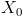. Then he flips a coin: tails he has a gain of 1, heads he experiences a loss of 1. At time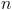, his wealth is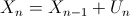where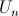is associated to theth coin:is equal to 1 with probability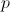(tails), and -1 with probability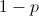(heads). It is also possible to write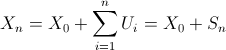where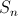can be interpreted as the net gain of the player. In order to get a good understanding of results that can be obtained. Assumeto be given. Let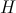denote the number of heads and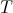the number of tails. Then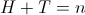, while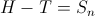. Let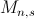denote the number of paths to go from point A (wealth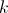at time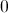) to point B (wealth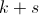at time). Note that this is a Markovian problem, that can be modeled using Markov chainsBut here, we will focus on combinatorial results. Hence,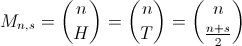In order to derive probabilities to reach, let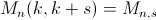denote the number of paths going from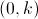to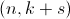. And let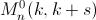denote the number of paths going fromtothat do reachat some point betweenand. Using a simple reflexion property, then ifandare positive,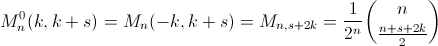Based on those reflexions, two results can be derived (focusing on probability, instead of counting paths). First, we can obtain that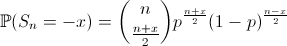(given that n and x have the same parity). The second result we can obtain is that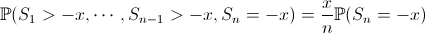Based on those two expressions, if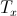denotes the first time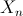become null, given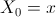,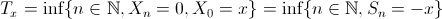then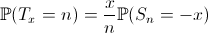This can be computed easily,

> x=10
> p=.55
> ProbN=function(n){
+ pb=0
+ if(abs(n-x) %% 2 == 0)
+ pb=x/n*choose(n,(n+x)/2)*(1-p)^((n+x)/2)*(p)^((n-x)/2)
+ return(pb)}
> plot(Vectorize(ProbN)(1:1000),type="s")That looks nice… But if we look closer, we can wonder what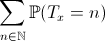would be ? Since we have the distribution of a probabilty measure, we might expect one. But here

> sum(Vectorize(ProbN)(1:1000))
 0.134385And this is not due to calculation mistakes that we do not get 1 here. Actually, we should write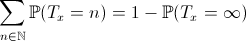which might be interpreted as the probability of ruin, starting from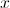, that we denote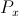from now on. The term on the left can be approximated using monte-carlo simulations

> p=.55
> x=10
> m=1000
> simul=10000
> S=sample(c(-1,1),size=m*simul,replace=TRUE,prob=c(1-p,p))
> MS=matrix(S,simul,m)
> for(k in 2:m) MS[,k]=MS[,k]+MS[,k-1]
> T0=function(vm) which(vm<=(-x))
> MTmin=apply(MS,1,T0)
> mean(is.na(MTmin)==FALSE)
 0.1328

To check the validity of the relationship above, a simple (theoretical) recursive formula can be derived for the term on the right (ruin probability), namely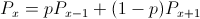with a boundary conditions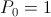, and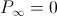. Then is comes that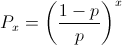Note that it might be tricky to check using monte carlo simulation… since we cannot have an infinite number of runs. And we’re dealing precisely with things that do occur when time is infinite. Actually, we can still check convergence, considering an upper limit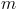for the number of runs, and then lettinggo to infinity. Note that an explicit formula can then be derived (using additional border condition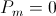)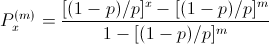Using the following code, it is possible to calculate ruin probability, in order to estimate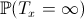.

> MSmin=apply(MS,1,min)
> mean(MSmin<=(-x))
 0.1328
> (((1-p)/p)^x-((1-p)/p)^m)/(1-((1-p)/p)^m)
 0.1344306

The following graph shows the evolution of ruin probability as a function of initial wealth (with monte carlo simulation, with a fixed horizon – including a confidence interval – versus the analytical expression)Hence, with stopping times, one should remember that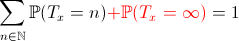and that those two terms can be approximated simply using simulations or standard approximations.

# Optimal reinsurance with ruin probability target

Exposé au 7th International Workshop on Rare Event Simulation, à l’IRISA à Rennes (RESIM’08). Les slides sont en ligne.

# Réassurance, solvavilité et probabilté

Exposé à Lyon dans le cadre du projet ANR AST& Risk.

In this talk, we consider optimal reinsurance from an insurer’s point of view. Given a (low) ruin probability target, the insurers wants to find the optimal risk transfer mechanism, i.e. either a proportional or a nonproportional reinsurance treaty. In the first case, a simple Monte Carlo algorithm can be designed, but in the nonproportional case, so far, no simple (and efficient) algorithm has been proposed